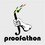# Homogeneity in Inequalities

I was reading this proof of 2001 IMO Problem #2, proving for $$a,b,c\in\mathbb{R}^+$$ that $$\sum \frac{a}{\sqrt{a^2+8bc}}\ge1$$. In a proof using Jensen's inequality, it says

This inequality is homogeneous so we can assume without loss of generality $a+b+c=1$.

I've read multiple proofs saying we can assume whatever because the terms are homogeneous, but what can you assume without loss of generality from homogeneity?Note by Cody Johnson
7 years ago

This discussion board is a place to discuss our Daily Challenges and the math and science related to those challenges. Explanations are more than just a solution — they should explain the steps and thinking strategies that you used to obtain the solution. Comments should further the discussion of math and science.

When posting on Brilliant:

• Use the emojis to react to an explanation, whether you're congratulating a job well done , or just really confused .
• Ask specific questions about the challenge or the steps in somebody's explanation. Well-posed questions can add a lot to the discussion, but posting "I don't understand!" doesn't help anyone.
• Try to contribute something new to the discussion, whether it is an extension, generalization or other idea related to the challenge.

MarkdownAppears as
*italics* or _italics_ italics
**bold** or __bold__ bold
- bulleted- list
• bulleted
• list
1. numbered2. list
1. numbered
2. list
Note: you must add a full line of space before and after lists for them to show up correctly
paragraph 1paragraph 2

paragraph 1

paragraph 2

[example link](https://brilliant.org)example link
> This is a quote
This is a quote
    # I indented these lines
# 4 spaces, and now they show
# up as a code block.

print "hello world"
# I indented these lines
# 4 spaces, and now they show
# up as a code block.

print "hello world"
MathAppears as
Remember to wrap math in $$ ... $$ or $ ... $ to ensure proper formatting.
2 \times 3 $2 \times 3$
2^{34} $2^{34}$
a_{i-1} $a_{i-1}$
\frac{2}{3} $\frac{2}{3}$
\sqrt{2} $\sqrt{2}$
\sum_{i=1}^3 $\sum_{i=1}^3$
\sin \theta $\sin \theta$
\boxed{123} $\boxed{123}$

Sort by:

In this post I consider only inequalities in three variables, but it extends to any number of variables. Expression $f(a,b,c)$ is said to be homogeneous of degree $k$ if and only if there exists real $k$ such that for every $t > 0$ we have $t^k \cdot f(a,b,c) = f(ta,tb,tc)$ For instance in your example we have $f(a,b,c) = \sum_{\text{cyc}} \frac{a}{\sqrt{a^2 + 8bc}} - 1$ and this expression $f$ is homogeneous of degree $0$, i.e. $f(a,b,c) = f(ta,tb,tc)$

Okay, now finally why can the assumption $a + b + c = 1$ be made? Assume that $a + b + c = m$ for $m > 0$ i.e. $\frac{a}{m} + \frac{b}{m} + \frac{c}{m} = 1$ Let $a' = \frac{a}{m}$, $b' = \frac{b}{m}$, $c' = \frac{c}{m}$. Then $a' + b' + c' = 1$ But the homegeneity of degree $0$ tells us that $f(a',b',c') = f\left(\frac{a}{m} + \frac{b}{m} + \frac{c}{m}\right) = f(a,b,c)$ (in case it's not clear, we used $\frac{1}{m} = t$, remember that $t$ can be arbitrary positive real number). Hence proving $f(a',b',c') \geq 0$ is equivalent to proving $f(a,b,c) \geq 0$ and we have the nice condition that $a' + b' + c' = 1$

You can assume many other things (but only one assumption at a time), like $a = 1$ $b = 1$ $c = 1$ $abc = 1$ $ab + bc + ca = 1$ $a^2 + b^2 + c^2 = 1$ etc. also the number on right-hand side of these assumptions doesn't need to be $1$.

- 7 years ago

Do you have any other good examples of how homogeneity is useful?

- 7 years ago

In general, homogeneous inequalities are easier to work with, that's because most known inequalities (AM-GM, Cauchy-Schwarz, etc.) are themselves homogeneous, so they are easier to apply to homogeneous inequalities, that's not to say that you can't prove non-homogeneous inequalities with them, but it's just usually easier to prove homogeneous inequalities.

Another reason is that many techniques rely on homogeneity such as SHED or [PDF] sum of squares

EDIT: Deleted example because it was wrong. (I am too tired.)

- 7 years ago

i got the above example but can u pls explain how it can be used in inequality like:

a^2/(a+1) + b^2/(b+1) >= 1/3, where a+b=1;

(i am getting K as 1, which is possible wrong)

- 5 years, 3 months ago

In this case you must homogenize (make it homogeneous) by taking $\frac{a^2}{a+1}=\frac{a^2}{(a+b)(2a+b)}$ and similar (hint: to finish, use Titus lemma)

- 5 years, 2 months ago

yes i did the same thing and solved the inequality. but i was trying to figure out K using the method described above. can you please tell the degree of homogeniety (K) in my problem.

i got K=1, but since this inequality is equivalent to : a^3 + b^3 >= ((a^2)b) + ((b^2)a), this gives K=3.

- 5 years, 2 months ago

Thank you so much!

- 7 years ago

What should I assume, if the given inequality is homogenous equation of degree one? @Jan J.

- 4 years, 9 months ago

But how can we assume $a=1$?!

- 2 years, 3 months ago

A symmetric equation can be assumed true.

- 6 years, 11 months ago

http://www.artofproblemsolving.com/Forum/viewtopic.php?f=52&t=386799&p=2148037#p2148037 in here

- 7 years ago